×#### Thank you for registering.

One of our academic counsellors will contact you within 1 working day.

Click to Chat

1800-1023-196

+91-120-4616500

CART 0

• 0

MY CART (5)

Use Coupon: CART20 and get 20% off on all online Study Material

ITEM
DETAILS
MRP
DISCOUNT
FINAL PRICE
Total Price: Rs.

There are no items in this cart.
Continue Shopping```Functions

Table of Content

What are Functions?

What is the domain of a Function? Also explain its co-domain and Range

Which is not the graph of a Function?

What are the Classification of Functions?

How to find Domain for any Functions?

What are the steps to find Range of any Functions?

What do you mean by Equal or Identical Function?

What are Periodic Functions?

What are Functions?Functions are the special class of relation or we can say that special types of relations are called as functions. It is the important concept used frequently in mathematics.

A function is like a machine which gives unique output for each input that is fed into it. But every machine is designed for certain defined inputs for e.g. a washing machine is designed for washing cloths and not the wood. Similarly, the functions are also defined for certain inputs which are called as its Domain and corresponding outputs are called Range.

Let A and B be two sets and let there exist a manner or rule or correspondence ‘f’  which associates evry element of A to a special or unique element in B, then f is called a Function or Mapping from A to B. It is denoted by symbol.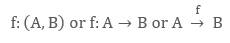Which reads ‘f is a function from A to B' or' f maps A to B.

If an elements a ∈ A is associated with an element b ∈ B then b is called ‘Image of a under f’ or ‘the f image of a’ or ‘the value of the function  at a’. Also a is called the pre – image of b or argument of b under the function we write it as

f : (a, b)  or f : a → b or b = f(a)

A relation  from a set A to a set B is called as the Function if it satisfies the below conditions:

All the elements of A should be mapped with the elements of B. That is, there should not be any element in A which is being unmapped with B. that is,  ∀a, (a, f(a))∈ f,where, a is the elements of set A.
Elements of set A should be uniquely mapped with the elements of set B. that is, if (a, b)∈ f & (a, c)∈ f, ⇒ b = c

Thus the ordered pairs of f must satisfy the property that each element of A appears in some ordered pair and no two ordered pairs have same first elements. See the below figures to understand the above points.Note: Every function is a relation but every relation is not necessarily a function.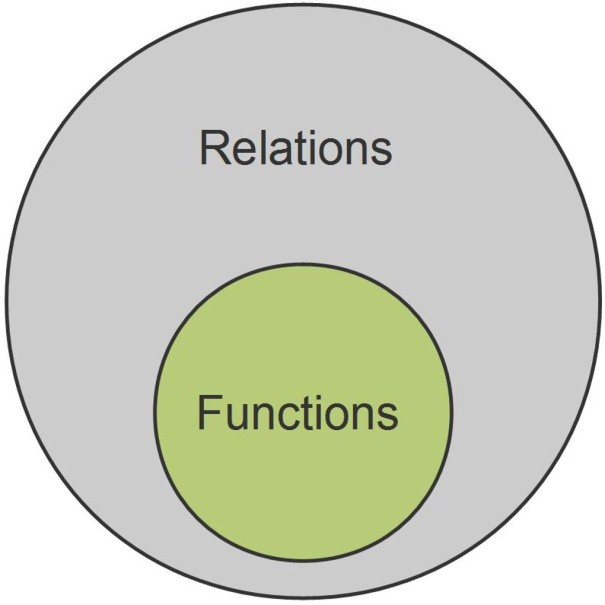What is the domain of a Function? Also explain its co-domain and Range

For a function from set A to set B that is, f(a, b), all the elements of set A are called as the Domain (or inputs) of the function f while all the elements of set B are called as the Co-domain (possible outputs) of the function f.

Range (is actual outputs) is the set or collection of all second elements from the ordered pairs (a, b) in the function f(a, b).

Domain of f = {a|a ∈ A, (a, f(a)) ∈ f}

Range of  f = {f(a)|a ∈ A, f (a) ∈ B, (a, f(a)) ∈ f}

For the function f(a, b), domain is considered as the input to function f while the co-domain is the possible outputs and range is the actual output.Similarly for a function, f: A → B, elements of set A are the inputs and B is the set of possible outputs. But the second elements of all orderedpair of f(A, B) will be the actual outputs.

It should be noted that range is a subset of co-domain. The Domain of the function whose functional equation or rule is given will be the set of those real numbers for which the function is defined. For a continuous function, range is represented in the interval form expressing minimum and maximum value.

Which is not the graph of a Function?

To identify any graph, weather it is a function or not, we must understand its definition once again but in terms of graphical meaning.

First condition of function says that – “All the elements of A should be mapped with the elements of B”. That means graphically, for every input in its domain, function must give or provide the corresponding output.

Second condition of the function says that – “Elements of set A should be uniquely mapped with the elements of set B”. These means that, for any input x, we must have one and only one output. The best way to check this condition for the function   is draw a line parallel to y – axis. If it cuts the graph at two or more distinct points, this means, for one value of x, we are getting more than one outputs. And hence it will not be a function.

Let’s take few functions and understand the concept of it graphically.

Example: y = x3

Here all the straight lines parallel to y – axis cut y = x3 only at one print.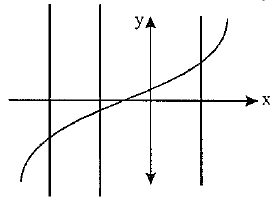Example: x2 + y2 = 1

Here line parallel to y –axis is intersecting the circle at two points hence it is not a function.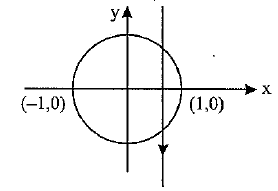What are the Classification of Functions?

Functions are classified as follows:

Polynomial Function:

If a function f is defined bywhere n is either 0 or positive integer but it can not be negative and an, an-1, …, a, a0. are real numbers and an ≠ 0 (otherwise the degree will not remain n), then f is called a Polynomial Function having degree n. A polynomial function comes under the category of continuous functions.

Algebraic Function:

A function f is called an algebraic function if it only involves algebraic operations such as addition, subtraction, multiplication, division and taking roots.

ExampleNote: All the polynomials are algebraic but converse is not true. Transcendental function are all the other functions which are not algebraic.

Fractional Rational Function:

A function of the form,where g(x) & h(x) are polynomials & h(x) ≠ 0. The set of real x such that  will be its domain.

Example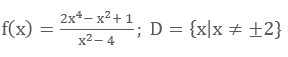Exponential Function:An Exponential Function is the function of the form f(x) = ax = exln⁡(a) (a >0, a ≠ 1, x ∈ R). f(x) = ax is defined as an exponential function as the variable x here is in the exponent. It should not be confused with power function g(x) = x2 in which variable x is the base. For  f(x) = ex domain in R and range is R+.

Logarithmic Function:

A function of the form y = loga⁡x, x > 0, a > 0, a ≠ 1, is called Logarithmic Function.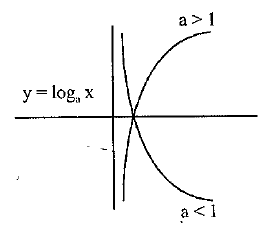Absolute Value Function (or Modulus Function)

An Absolute or Modulus Function is represented as y =  f(x) = |x|  and is read as mod or modulus of x. It is defined as: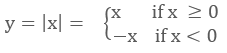For f(x) = |x|, domain is R and Range is R+ U {0}.

Signum Function: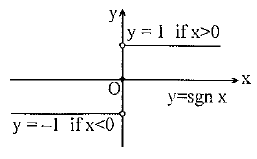A signum function y = f(x) = sgn(x) is defined as:It is also written asGreatest Integer Or Step up Function:

The function y = f(x) = [x] is called the Greatest Integer or Set up Function where  represents the greatest integer less than or equal to x.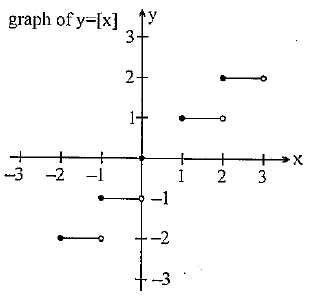Note that for:

-1 ≤ x < 0;  [x] = -1

0 ≤ x < 1;  [x] = 0

1 ≤ x <2;  [x] = 1

2 ≤ x <3; [x] = 2 and so on.

For f(x) = [x], domain is R and range is I.

Identity Function:

The f: A → B defined by f(x) = x , ∀ x ∈ A is called the Identity of A and is denoted by IA. The domain and range of identity function is entire real range that is, R

f(x) = x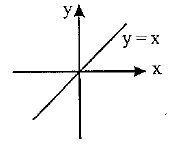Constant Function: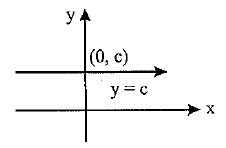The function f: A → B is said to be a constant function if its output is unaffected with its inputs that is, constant functions has fixed single (or singleton) range. Also, we may define as every element of A has the same f image in B. Thus  f: A → B; f(x) = c, ∀ x ∈ A, c ∈ B is a constant function. Note that the constant function may be one – one or many – one, onto or into.

Example

f(x) = [{x}]; g(x) = sin2⁡x + cos2⁡x; h(x) = sgn(x2 - 3x + 4) etc, all are constant functions.

How to find Domain for any Functions?

Finding domain is an easy task, we just need to take care of few points. So just note the below points,Expression under even root (that is, square root, fourth root, sixth root etc) should be ≥ 0.

Denominator should not be zero (D ≠ 0).

For logb⁡ x, x > 0 & b > 0 (b ≠ 1)

If  D1 & D2 domains of y = f(x) & y = g(x) respectively, then the domain of f(x) ± g(x) or f(x). g(x) is D1⋂ D2

For f(x)/g(x) domain is D1 ⋂ D2 - {g(x) = 0}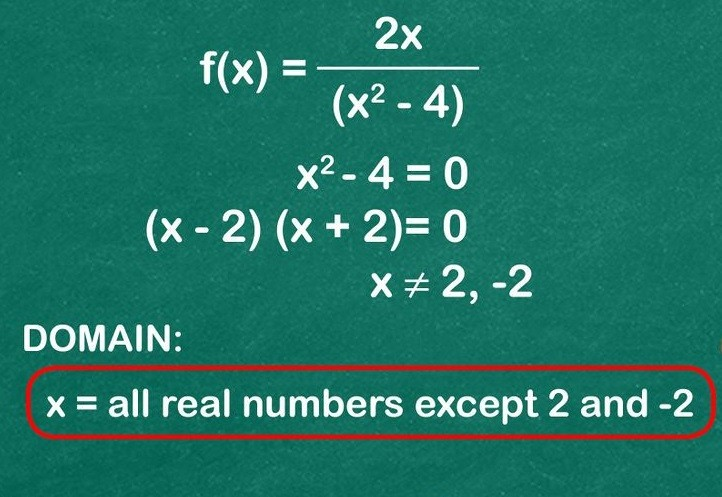What are the steps to find Range of any Functions?

Range of any function y= f(x) is the collection or set of all outputs corresponding to each real number in its domain.

We need to follow below steps to find the range of function.

First, find the domain of y = f(x)

If the domain has finite number of elements, then range is the set of corresponding output or f(x) values.

While if domain has infinite elements or R or R- {some finite points}, then express x in terms of y. Then solve the equation and find x such that y is real.

Example

Find the Range of y = 3 – 2x

Domain is x ∈ R

0 ≤ 2x < ∞

Range ∈ (-∞, 3)

What do you mean by Equal or Identical Function?

Two functions (say g(x) and h(x)) are said to be equal if they satisfy below points:

The domain of g = the domain of h

The Range of g = range of h

g(x) = h(x), for every x belonging to their common domain

Example

g(x) = 1/x & h(x) =x/x2 are identical functions.

Graphically, two functions are equal if their graphs are identical or same.

What are Periodic Functions?

A function f(x) is periodic if its outputs start repeating after every fixed interval. Let this fixed interval is presented by a positive number P (P >0) such that f(x + T) = f(x),  for all values of x within the domain of x. This positive number P, is called as the Period of the Function.

Example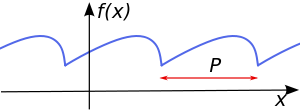Sine and Cosine functions both are periodic with period 2π and tan x is periodic with period π.

Graphically, it is more easy to understand and conclude the periodic functions. The graph of the periodic function repeats after every fixed interval. That is, for periodic functions, repetition in graph can be easily seen. This quantitative repetition or fixed interval is called as the Period of the Function which is the width of the interval.

Important Properties:

f(P) = f(0)= f(-P), where P is the period.

Inverse of a periodic function does not exist.

Every constant function is always periodic, with no fundamental period.

If f(x) has a period P, then 1/f(x) and √f(x) also has a period p.

If f(x) has a period P, then f(ax + b) has a period T/|a|

If f(x) has a period P and g(x) also has a period P, then it does not mean that f(x) + g(x) must have a period P.

If f(x) and g(x) are periodic then f(x) + g(x) need not be periodic.

Example

f(x) = cos x and g(x) = {x}

Function
```### Course Features

• 731 Video Lectures
• Revision Notes
• Previous Year Papers
• Mind Map
• Study Planner
• NCERT Solutions
• Discussion Forum
• Test paper with Video Solution